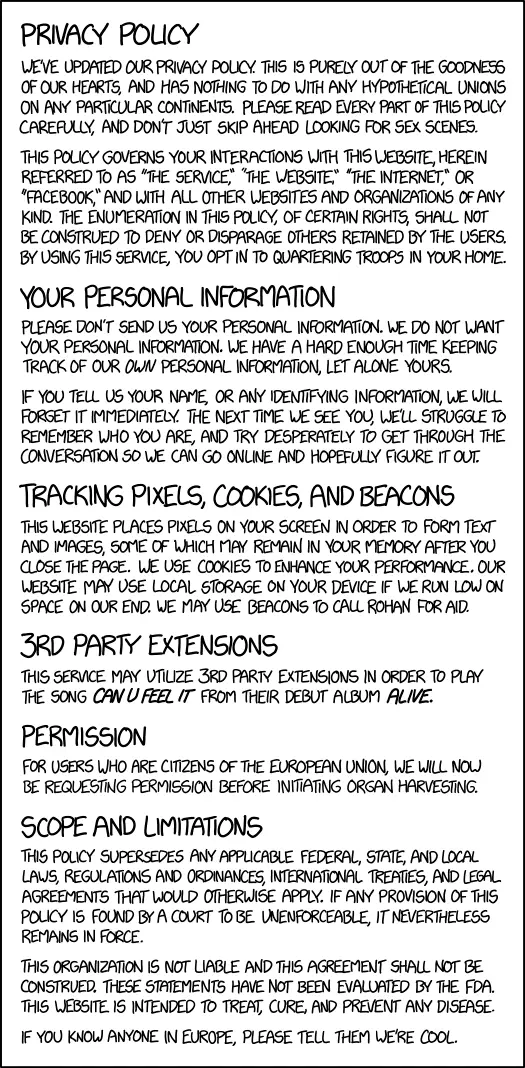# slama.dev

I don’t use your personal data. I mean… why would I? This is a personal blog, what would I even do with it? However, since all cool modern websites have them, I must too, so here is a filled-in template.

### Definitions

Since I don’t really have anything to talk about in this section but the template includes it, here are some definitions that I think are useful. Feel free to thank me later.

• the function $f$ has a total differential in the point $\mathbf{a}$, if there exists a continuous function $\mu$ in the neighbourhood of $U$ of the point $\mathbf{o} \in \mathbb{R}^n$, such that $\mu(\mathbf{o}) = 0$, and numbers $A_1, \ldots, A_n$ for which the following holds: $f(\mathbf{a} + \mathbf{h}) - f(\mathbf{a}) = \sum_{k - 1}^{n} A_k h_k + || \mathbf{h} || \mu(\mathbf{h})$
• the Tutte polynomial of a multigraph $G = (V, E)$ is a polynomial of $x, y$ defined as $T_G(x, y) = \sum_{F \subseteq E} (x - 1)^{r(E) - r(F)} \cdot (y - 1)^{n(F)}$
• a mitochondria is a double-membrane-bound organelle found in most eukaryotic organisms

### Collecting and using your personal data

No.

#### Retention of your personal data

I will not retain your personal data only for as long as you want me to. If you feel like it is necessarry for me to have it, feel free to email it to my email adress (tomas [at] slama [dot] dev).

#### Disclosure of your personal data

I pinky promise not to share the fact that I don’t have any of your personal data with anyone. There, I pinky promised, that should suffice in any reasonable court of law.